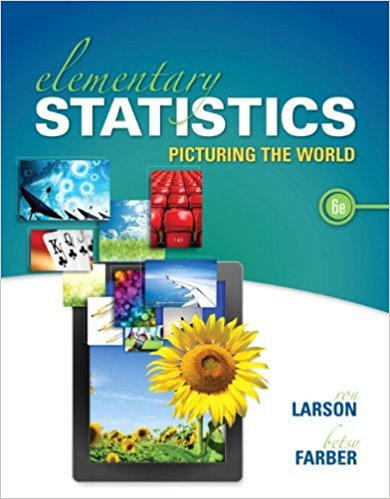×
Log in to StudySoup
Get Full Access to Elementary Statistics: Picturing The World - 6 Edition - Chapter 5.r - Problem 70e
Join StudySoup for FREE
Get Full Access to Elementary Statistics: Picturing The World - 6 Edition - Chapter 5.r - Problem 70e

Already have an account? Login here
×
Reset your password

# In Exercise, determine whether you can use a normalISBN: 9780321911216 66

## Solution for problem 70E Chapter 5.R

Elementary Statistics: Picturing the World | 6th Edition

• Textbook Solutions
• 2901 Step-by-step solutions solved by professors and subject experts
• Get 24/7 help from StudySoup virtual teaching assistantsElementary Statistics: Picturing the World | 6th Edition

4 5 1 428 Reviews
16
4
Problem 70E

Problem 70E

In Exercise, determine whether you can use a normal distribution to approximate the binomial distribution. If you can, use the normal distribution to approximate the indicated probabilities and sketch their graphs. If you cannot, explain why and use a binomial distribution to find the indicated probabilities.

Thirty-one percent of people in the United States have type A+ blood. You randomly select 40 people in the United States and ask them whether their blood type is A+. Find the probability that the number of people who have A+ blood is (a) exactly 15, (b) less than 10, and (c) between 20 and 35, and (d) identify any unusual events. Explain.

Step-by-Step Solution:

Solution:

Step 1 of 5:

Given in a survey, 31% of U.S. have A+ blood group.

If we randomly select 40 U.S. people and ask them whether their blood group is A+.

If a Binomial distribution is approximated by a Normal distribution if np and nq are greater than or equal to 5.

n = 40 and p = 0.31.

np = 40(0.31)

= 12.4 and

nq = 40(1 - 0.31)

= 27.6.

Both np and nq are greater than 5.

Step 2 of 5

Step 3 of 5

##### ISBN: 9780321911216

Unlock Textbook Solution

Enter your email below to unlock your verified solution to:

In Exercise, determine whether you can use a normal